Next: Matrix decompositions Up: Matrix decompositions and latent Previous: Matrix decompositions and latent   Contents   Index

# Linear algebra review

We briefly review some necessary background in linear algebra. Let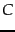be an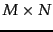matrix with real-valued entries; for a term-document matrix, all entries are in fact non-negative.

The rank of a matrix is the number of linearly independent rows (or columns) in it; thus,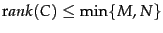. A square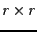matrix all of whose off-diagonal entries are zero is called a diagonal matrix; its rank is equal to the number of non-zero diagonal entries. If all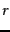diagonal entries of such a diagonal matrix are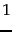, it is called the identity matrix of dimensionand represented by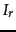.

For a square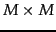matrixand a vector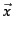that is not all zeros, the values of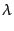satisfying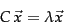(213)

are called the eigenvalues of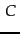. The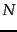-vectorsatisfying Equation 213 for an eigenvalueis the corresponding right eigenvector. The eigenvector corresponding to the eigenvalue of largest magnitude is called the principal eigenvector. In a similar fashion, the left eigenvectors ofare the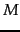-vectors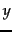such that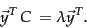(214)

The number of non-zero eigenvalues ofis at most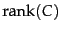.

The eigenvalues of a matrix are found by solving the characteristic equation, which is obtained by rewriting Equation 213 in the form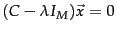. The eigenvalues ofare then the solutions of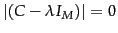, where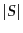denotes the determinant of a square matrix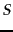. The equationis anth order polynomial equation inand can have at mostroots, which are the eigenvalues of. These eigenvalues can in general be complex, even if all entries ofare real.

We now examine some further properties of eigenvalues and eigenvectors, to set up the central idea of singular value decompositions in Section 18.2 below. First, we look at the relationship between matrix-vector multiplication and eigenvalues.

Worked example. Consider the matrix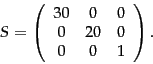(215)

Clearly the matrix has rank 3, and has 3 non-zero eigenvalues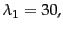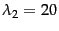and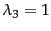, with the three corresponding eigenvectors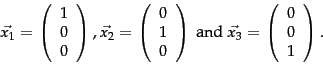(216)

For each of the eigenvectors, multiplication byacts as if we were multiplying the eigenvector by a multiple of the identity matrix; the multiple is different for each eigenvector. Now, consider an arbitrary vector, such as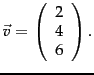We can always express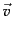as a linear combination of the three eigenvectors of; in the current example we have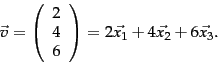(217)

Suppose we multiplyby: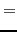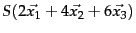(218)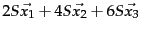(219)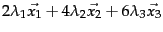(220)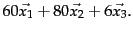(221)

End worked example.

Example 18.1 shows that even thoughis an arbitrary vector, the effect of multiplication byis determined by the eigenvalues and eigenvectors of. Furthermore, it is intuitively apparent from Equation 221 that the product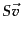is relatively unaffected by terms arising from the small eigenvalues of; in our example, since, the contribution of the third term on the right hand side of Equation 221 is small. In fact, if we were to completely ignore the contribution in Equation 221 from the third eigenvector corresponding to, then the productwould be computed to be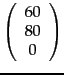rather than the correct product which is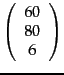; these two vectors are relatively close to each other by any of various metrics one could apply (such as the length of their vector difference).

This suggests that the effect of small eigenvalues (and their eigenvectors) on a matrix-vector product is small. We will carry forward this intuition when studying matrix decompositions and low-rank approximations in Section 18.2 . Before doing so, we examine the eigenvectors and eigenvalues of special forms of matrices that will be of particular interest to us.

For a symmetric matrix, the eigenvectors corresponding to distinct eigenvalues are orthogonal. Further, ifis both real and symmetric, the eigenvalues are all real.

Worked example. Consider the real, symmetric matrix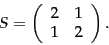(222)

From the characteristic equation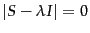, we have the quadratic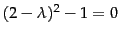, whose solutions yield the eigenvalues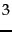and. The corresponding eigenvectors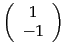and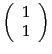are orthogonal. End worked example.

SubsectionsNext: Matrix decompositions Up: Matrix decompositions and latent Previous: Matrix decompositions and latent   Contents   Index